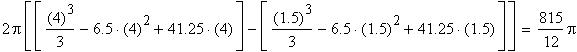Problem
Find the volume of the solid generated by rotating the region bounded by y = x, y = 3 – x, and x = 4 around the line x = 5.
Solution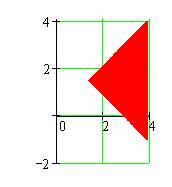When rotated around the line x = 5, region R generates this shape: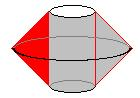Since this shape is symmetric around the line y = 1.5, we'll set up the integral to find the volume of the upper half, and double it.
Horizontal cross sections of the shape are washers with inner radius 1 and varying outer radii. For washers above y = 1.5, the outer radius for a given value of y is the horizontal distance between a point P on the outer radius on the line y = x and the line x = 5. The distance inward from the largest outer radius (where x = 1.5) is the same as the distance y above 1.5, so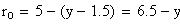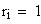The volume of the shape is the infinite sum of areas of washers with thickness Dy, given by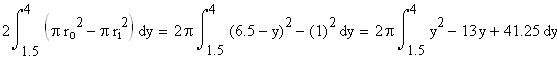This evaluates to